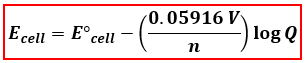# Problem: What would the potential of a standard hydrogen (S.H.E.) electrode be if it was under the following conditions?[H+] = 0.77 MPH2 = 1.4 atmT = 298 K

###### FREE Expert Solution

The Nernst Equation at 25°C or 298 K:Ecell = cell potential under non-standard conditions
cell = standard cell potential
n = number of e- transferred
Q= reaction quotient = [products]/[reactants]###### Problem Details

What would the potential of a standard hydrogen (S.H.E.) electrode be if it was under the following conditions?

[H+] = 0.77 M

PH2 = 1.4 atm

T = 298 K